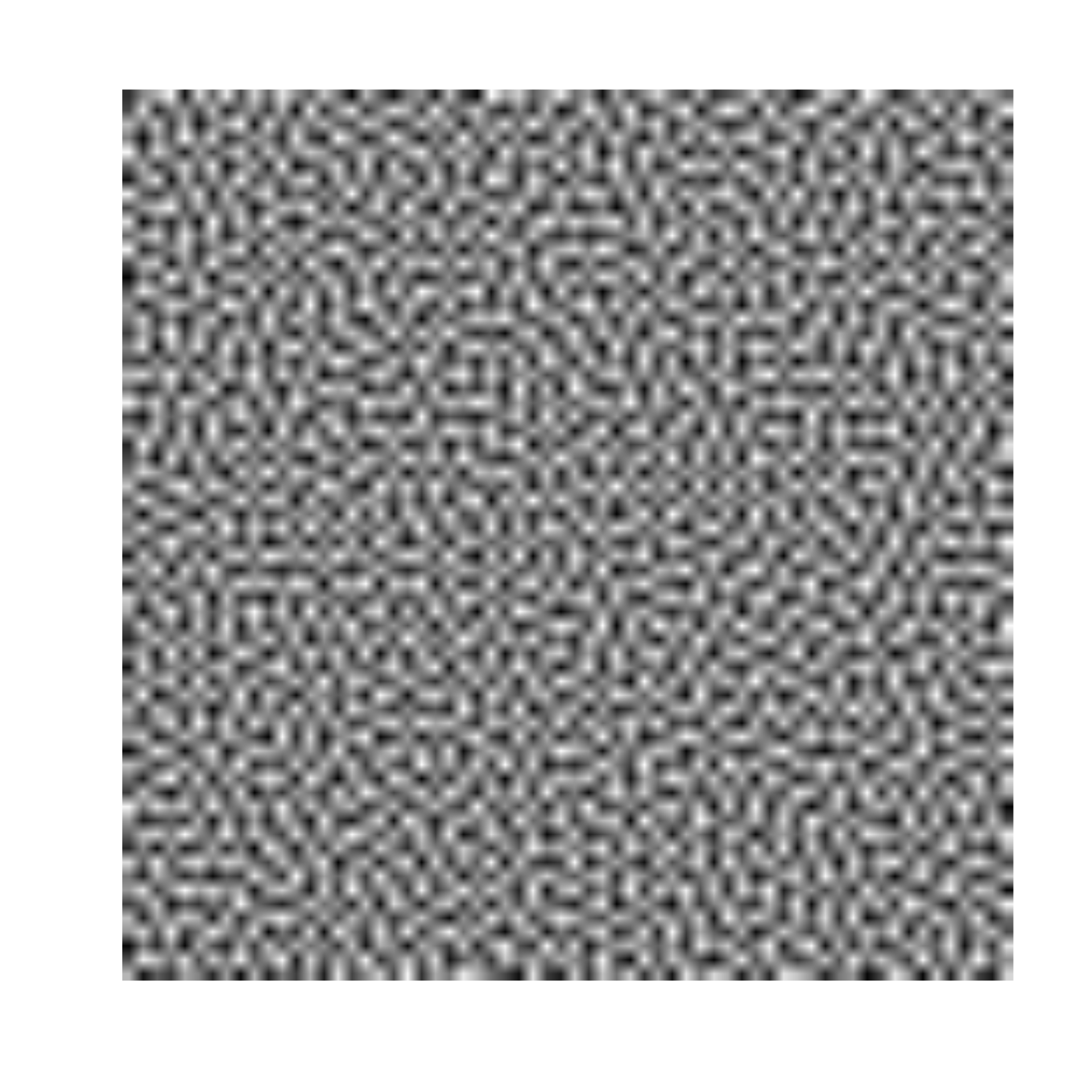Blue noise is a form of noise that has weak low-frequency. This means that it is devoid of larger structures and can be blurred to an even gray. Blue noise in ambient is calculated using the popular Void-and-cluster method developed by Ulichney. Calculating blue noise is much more computationally expensive than e.g. white noise so ambient does not provide a gen_blue() generator, only the noise_blue() texture function. Computation time increases linearly with the number of pixels in the texture and can get prohibitly long very soon. However, blue noise is tile-able so a good suggestion is to try tiling e.g. a 64x64 texture to the desired dimensions and see if that suffices.

noise_blue(dim, sd = 10, seed_frac = 0.1)

## Arguments

dim

The dimensions (height, width, (and depth, (and time))) of the noise to be generated. The length determines the dimensionality of the noise.

sd

The standard deviation of the gaussian filter to apply during the search for clusters and voids.

seed_frac

The fraction of pixels to seed the algorithm with during start

## Value

For noise_white() a vector if length(dim) == 1, matrix if length(dim) == 2 or an array if length(dim) >= 3.

## Examples

# Basic use
noise <- noise_blue(c(64, 64))

plot(as.raster(normalise(noise)))